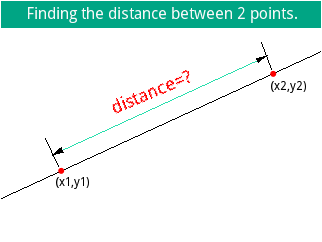Rotate Text Help!
10-31-2016, 05:50 AM (This post was last modified: 10-31-2016 03:18 PM by Spybot.)
Post: #1SpybotMember Posts: 178 Joined: Feb 2015
Rotate Text Help!
Hello!

I'm trying to make a drawing in the Prime, but I realize I can't rotate texts!
I just wanted to rotate the "distance=?" text a couple of degrees, so it aligns with the green line (screen shot).

Here's my code:

BEGIN
RECT();
DIMGROB_P(G1,320,240);
RECT_P(G1,RGB(255,255,255));
LINE_P(G1,0,197,320,50,RGB(0,0,0));
LINE_P(G1,52,153,265,55,RGB(40,220,170));
LINE_P(G1,57,165,44,137,RGB(0,0,0));
LINE_P(G1,270,68,260,41,RGB(0,0,0));
RECT_P(G1,0,0,320,26,RGB(0,160,128));
TEXTOUT_P("Finding the distance between 2 points.",G1,25,2,4,RGB(255,255,255));

TEXTOUT_P("(x1,y1)",G1,55,175,2,RGB(0,0,0));
TEXTOUT_P("(x2,y2)",G1,270,79,2,RGB(0,0,0));
TEXTOUT_P("●",G1,56,162,3,RGB(255,0,0));
TEXTOUT_P("●",G1,268,65,3,RGB(255,0,0));
TEXTOUT_P("distance=?",G1,100,85,5,RGB(255,0,0));//this one I need to rotate.

TRIANGLE_P(G1,255,57,257,61,266,54,RGB(0,0,0));
TRIANGLE_P(G1,62,151,51,154,60,146,RGB(0,0,0));
BLIT_P(G0,G1);
FREEZE;
END;

I'll appreciate any help, Thank you.

Attached File(s) Thumbnail(s)Spybot.
10-31-2016, 05:33 PM
Post: #2eriedSenior Member Posts: 744 Joined: Dec 2013
RE: Rotate Text Help!
To rotate something, simplest way is to use a translation matrix (i.e. with xyz vectors). Of course you can use a simpler form if you don't consider the axis of the rotation (make it 0) and remove z:
Code:
local mat:=[[cos(angle),sin(angle)],[-sin(angle),cos(angle)]];

The new position of every rotated pixel will be equal to:
Code:
newpos:=mat*[[sourceX],[sourceY]];

Working code:
Code:
RotateG(source,destination,angle) BEGIN DIMGROB(destination,320,240); // Rotate matrix local tmp;local mat:=[[cos(angle),sin(angle)],[-sin(angle),cos(angle)]]; FOR X FROM 0 TO grobh(source) DO FOR Y FROM 0 TO grobw(source) DO tmp:=mat*[[X],[Y]]; PIXON_P(destination,X,Y,GETPIX_P(source,tmp(1,1),tmp(2,1))); end; end; END; EXPORT AboutESP() BEGIN RECT(); DIMGROB_P(G1,320,240); RECT_P(G1,RGB(255,255,255)); LINE_P(G1,0,197,320,50,RGB(0,0,0)); LINE_P(G1,52,153,265,55,RGB(40,220,170)); LINE_P(G1,57,165,44,137,RGB(0,0,0)); LINE_P(G1,270,68,260,41,RGB(0,0,0)); RECT_P(G1,0,0,320,26,RGB(0,160,128)); TEXTOUT_P("Finding the distance between 2 points.",G1,25,2,4,RGB(255,255,255)); TEXTOUT_P("(Straight Line Kit)",G1,95,30,4,RGB(255,255,255)); TEXTOUT_P("(x1,y1)",G1,55,175,2,RGB(0,0,0)); TEXTOUT_P("(x2,y2)",G1,270,79,2,RGB(0,0,0)); TEXTOUT_P("●",G1,56,162,3,RGB(255,0,0)); TEXTOUT_P("●",G1,268,65,3,RGB(255,0,0)); // ----------------------------- DIMGROB(G3,320,240); // temporal TEXTOUT_P("distance=?",G3,60,130,5,RGB(255,0,0));//this one I need to rotate. RotateG(G3,G2,-0.4); // Rotate 0.4 radians BLIT_P(G1,G2,RGB(255,255,255)); // Transparency // ----------------------------- TRIANGLE_P(G1,255,57,257,61,266,54,RGB(0,0,0)); TRIANGLE_P(G1,62,151,51,154,60,146,RGB(0,0,0)); BLIT_P(G0,G1); FREEZE; END;Of course this code is not very efficient, you may crop the source to size, add the "axis" for the rotation, etc...

My website: erwin.ried.cl
11-01-2016, 06:43 AM
Post: #3SpybotMember Posts: 178 Joined: Feb 2015
RE: Rotate Text Help!
I wasn't aware of what you showed here, I just thought rotating things was a simpler thing to do, I see this is a little bit more advanced than I thought, but I will review this code until I get a deeper understanding of it.

Spybot.
11-02-2016, 04:12 PM (This post was last modified: 11-04-2016 02:20 PM by Chasfield.)
Post: #4
 ChasfieldMember Posts: 119 Joined: Sep 2015
RE: Rotate Text Help!
I think Eried's approach is the best you will get - generate the pixels with the available TEXTOUT_P command then rotate the pixel field on screen.

A wackier option would be to build text characters out of LINE_P statements - making a sort of vector font. Tedious, but you can get there in the end.

Attached, as an example, is a circular, on-screen slide rule I coded in Borland Delphi many years ago using this approach.

I defined blocky but serviceable numeric characters using Delphi line drawing statements. I seem to remember that rotating these vector elements to animate the rule and its scales then wasn't too difficult.

NB, I think the slide rule runs fine on newer versions of Windows but the window might be sizeable, even though it was defined to be of fixed size in Delphi. This spoils the show a bit. Anyway, It runs as designed on my Linux/Wine set-up.

Attached File(s)SlideR.zip (Size: 103.4 KB / Downloads: 9)
 « Next Oldest | Next Newest »

User(s) browsing this thread: 1 Guest(s)